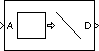# Extract Diagonal

Extract main diagonal of input matrix

•Libraries:

## Description

The Extract Diagonal block populates the unoriented output vector with the elements on the main diagonal of the M-by-N input matrix `A`. Equivalent MATLAB® code is given by:

`D = diag(A) `

## Ports

### Input

expand all

Input matrix to extract the diagonal from, specified as a scalar, vector, or matrix .

Data Types: `single` | `double` | `int8` | `int16` | `int32` | `uint8` | `uint16` | `uint32` | `Boolean` | `fixed point`
Complex Number Support: Yes

### Output

expand all

Output vector of each diagonal element.

The output is the same data type and complexity as the input. The output vector has length `min(M,N)`.

Data Types: `single` | `double` | `int8` | `int16` | `int32` | `uint8` | `uint16` | `uint32` | `Boolean` | `fixed point`
Complex Number Support: Yes

## Block Characteristics

 Data Types `Boolean` | `double` | `fixed point` | `integer` | `single` Direct Feedthrough `no` Multidimensional Signals `no` Variable-Size Signals `no` Zero-Crossing Detection `no`

## Version History

Introduced before R2006a

expand all